﻿ Application of Grey System Theory to Assessment of Computational Thinking SkillsPublications are Open
Access in this journal
Article Versions
Export Article
• Normal Style
• MLA Style
• APA Style
• Chicago Style
Research Article
Open Access Peer-reviewed

### Application of Grey System Theory to Assessment of Computational Thinking Skills

Michael Gr. Voskoglou
American Journal of Applied Mathematics and Statistics. 2018, 6(6), 253-261. DOI: 10.12691/ajams-6-6-6
Received October 20, 2018; Revised November 01, 2018; Accepted November 30, 2018

### Abstract

Computational thinking is a kind of analytic thinking that synthesizes critical thinking and existing knowledge and applies them for solving complex real life and technological problems, designing systems, and understanding human behaviour, by drawing on fundamental principles of computer science. This involves frequently a degree of uncertainty and (or) the use of approximate data. On the other hand, a grey system is characterized by lack of adequate information about its components and (or) its function and the corresponding theory has found nowadays many applications to real life, science and engineering. In grey system theory the main tool for handling approximate data is the use of the grey numbers, which are indeterminate numbers defined with the help of the closed real intervals. In the present work grey numbers are used for evaluating computational thinking skills and examples are presented to illustrate our results. The outcomes of this new assessment method are compared to the corresponding outcomes of the classical method of calculating the GPA index and of a similar method developed in earlier works that uses as tools triangular fuzzy numbers instead of grey numbers.

### 1. Introduction

An organised system is defined to be a set of interacting components forming an integrated whole and working together for achieving a common target. A factory, a hospital, a bank, etc. are common examples of systems, whereas in general one can distinguish between physical, biological, social, economic, engineering, abstract knowledge systems, etc. As a multi-perspective domain systems’ theory serves as a bridge for an interdisciplinary dialogue between autonomous areas of study 1. In the present work we deal with Computational Thinking (CT) systems.

The assessment of a system’s performance is a very important part of the systems’ theory, because it enables the system’s designer to correct its weaknesses and therefore to increase its effectiveness. When the performance of a system’s components is evaluated with numerical scores, then the traditional way for assessing the system’s mean performance is the calculation of the mean value of those scores. However, in order to comfort the user’s existing uncertainty about the exact value of the numerical scores corresponding to each of the system’s components, frequently in practice the assessment is made not by numerical scores but by qualitative linguistic expressions, like excellent, very good, good, etc, which makes the calculation of their mean value impossible.

A popular in such cases method for evaluating the overall system’s performance is the calculation of the Grade Point Average (GPA) index ( 2, Chapter 6, p.125). However, GPA is a weighted average in which greater coefficients (weights) are assigned to the higher grades, which means that it reflects not the mean, as we wish, but the quality system’s performance.

In order to overcome this difficulty we have utilized in earlier works the system’s total uncertainty under fuzzy conditions (due to the qualitative assessment of its components) as a measure of its effectiveness ( 2, Chapter 5). This manipulation is based on a fundamental principle of the Information Theory according to which the reduction of a system’s uncertainty is connected to the increase of information obtained by a system’s activity. In other words, lower uncertainty indicates a greater amount of information and therefore a better system’s performance with respect to the corresponding activity. However, this method needs laborious calculations, cannot give a precise qualitative characterization of a system’s performance and, most importantly, it is applicable for comparing the performance of two different systems with respect to a common activity only under the assumption that the existing uncertainty is the same in the two systems before the activity. This was the reason for turning in later works our attention to the use of Fuzzy Numbers (FNs) for the assessment of a system’s mean performance under fuzzy conditions ( 2, Chapter 7).

In the present work Grey Numbers (GNs) are used for assessing the performance of CT systems, in which vagueness and/or uncertainty are frequently appearing. This method is proved to be equivalent to the method using a special form of FNs, the Triangular FNs (TFNs) (e.g. see 3), but it has the advantage of reducing significantly the required computational burden. Moreover, the GNs are defined easily with the help of the closed intervals of real numbers, in contrast to the TFNs that need the knowledge of basic elements of the theory of Fuzzy Sets (FS).

The rest of the paper is formulated as follows: Section 2 is devoted to a brief description of the CT process and of the Case-Based Reasoning (CBR) systems that are special forms of CT systems. In Section 3 the assessment method with the TFNs is briefly recalled and the background information is given about GNs, needed for the understanding of the article. The new assessment method using GNs is developed in Section 4 and its equivalence to the method with TFNs is also proved. Examples with CT situations illustrating those methods are presented in Section 5. The article closes with our conclusions and a short discussion on the perspectives of future research on the subject, presented in Section 6.

### 2. Computational Thinking

2.1 Computational and Critical Thinking in Problem Solving

The importance of Problem Solving (PS) has been realised for such a long time that in a direct or indirect way affects our daily lives for ages. However, even graduates have nowadays difficulty in solving real life problems. Somehow, they can not apply theory into practice, or theorise/reflect on practice 4. In fact, it is the human mind at the end that has to be applied in a problematic situation and its capacity to solve the problem is directly related to the knowledge stored in the mind. But knowledge is the product of thinking that varies from a very simple and mundane thought to a very sophisticated and complex one. The nature of a problem dictates the level of thinking required to solve it.

A simple problem can be solved by applying a lower-order thinking that requires minimum cognitive effort. On the contrary, the solution of composite non-routine problems requires a higher-order thinking that is referred as Critical Thinking (CrT) 5. CrT has its roots to the ancient Greek philosopher Socrates, who perfected the art of questioning, whereby by asking pertinent questions he would show that “people could not rationally justify their confident claims of knowledge”. CrT can be conceptualised as a complex mode of thinking involving abstraction, uncertainty, application of multiple criteria, reflection, self-regulation, making judgements, analysis and synthesis and so on 6. Such kind of thinking, which often generates multiple solutions, facilitates the transfer of knowledge, i.e. the use and transformation of already existing knowledge in creating new knowledge. In concluding, creativity and innovation driven by tacit knowledge and CrT driven by logic become the necessary tools for solving non-routine problems.

However, living in a knowledge era and in an ever increasing progress in technology, combining knowledge and technology to solve problems becomes the mode rather than the exception. In an attempt by humans to increase the power of the mind (Artificial Intelligence), the holder of a Nobel prize Herbet Simon saw thinking as information-processing and computers started taking over as a kind of ‘thinking machines’ 7. If technology is added as a tool, then Computational Thinking (CT) is another essential component of thinking required for solving complex real life or technological problems. The term CT was first introduced by S. Papert 8, who is widely known as the “father” of the Logo software. However, it was brought to the forefront of the computer society by Wing 9 to describe how to think like a computer scientist. Wing has described CT as “solving problems, designing systems and understanding human behaviour by drawing on concepts fundamental to computer science”.

The main characteristics of CT include:

Ÿ Analyzing and logically organizing data

Ÿ Data modelling, data abstractions, and simulations

Ÿ Formulating problems such that computers may assist

Ÿ Identifying, testing, and implementing possible solutions

Ÿ Automating solutions via algorithmic thinking

Ÿ Generalizing and applying this process to other problems (http://en.wikipedia.org/wiki/Computational-thinking)

It can be concluded that CrT is a prerequisite to knowledge acquisition and application to solve problems, but not a sufficient condition when we are faced with complex real life or technological problems, the solution of which requires also a pragmatic way of thinking such as CT. The way in which CrT and CT are simultaneously acting for the solution of such complex problems has not been clearly established yet. In an earlier work 10 we have attempted at shedding some light into this action. According to our approach, which is graphically represented in Figure 1, the given problem is considered as an obstacle to be passed through. The three cognitive components acting during the PS process are the already existing knowledge, CrT and CT. Our hypothesis is that, once awareness of the problem is made, the existing information is extracted and critically analyzed. Then, if there exists sufficient background knowledge, the necessary new knowledge is retrieved through CrT and the solver, thinking in a computer like scientific way (CT), applies that knowledge to solve the problem. In other words, CT synthesizes CrT and existing knowledge and applies them for the solution of the problem. This approach could be used as a model for the process of solving complex real life or technological problems.

• Figure 1. Voskoglou and Buckley’s problem solving model

According to Liu & Wang 11 CT is a hybrid of abstract, algorithmic, logical, constructive and modelling thinking. The last one refers to the translation of objects or phenomena from the real world into mathematical equations (mathematical models) or computer relations (simulation models). One could claim that modelling thinking constitutes the essence of CT, since it synthesizes all the modes of thinking involved in CT to construct a model for the solution of the corresponding problem.

Computer Science is not just about programming, it is about an entire way of thinking, which has become nowadays an intrinsic part of our lives. Computation is an increasingly essential tool for doing scientific research. It is expected that future generations of scientists and engineers will need to engage and understand computing in order to work effectively with scientific objects, technologies and methodologies. Nevertheless, CT builds on the power and limits of computing processes whether they are executed by a human or by a machine. Therefore, it is a fundamental skill to everyone, not just for scientists and engineers. To reading, writing and arithmetic, we should add CT to every child’s analytical ability 9.

It is widely accepted that motivation and involvement are imperative in retaining students in computer science and in order to do so we need to suitably engage students in the process of learning programming by building more effective mechanisms and tools for the development of programming skills. However, this is not an easy task. One of the core aims of learning programming should be to constantly highlight that programming is not only coding, but also thinking computationally and acquiring skills to develop solid solutions through understanding of concrete problems. Recent studies in this field address the necessity to become trained in thinking computationally before learning programming. They conclude that the education of programming along with the theory of computing needs to be represented in a way that would make sense to students within the computer science discipline 12.

2.2 Case-Based Reasoning Systems

Case-Based Reasoning (CBR) is the process of solving new problems by adapting the solution of similar (analogous) problems solved in the past, which are referred as past cases. A case-library can be a powerful corporate resource allowing everyone in an organization to tap in it when handling a new problem. A CBR system, usually designed and functioning with the help of computers, allows the case-library to be developed incrementally, while its maintenance is relatively easy and can be carried out by domain experts. It becomes evident that the greatest part of the CBR process involves CT, which means that the CBR systems constitute a special case of CT systems. The CBR approach has got a lot of attention over the last 30-40 years, because as an intelligent-systems method enables information managers to increase efficiency and reduce cost by substantially automating processes.

CBR has been formalized for purposes of computer and human reasoning as a four step process, often referred as the “four R’s”. These steps involve:

Ÿ R1: Retrieve the most similar to the new problem past case.

Ÿ R2: Reuse the information and knowledge of the retrieved case for designing the solution of the new problem.

Ÿ R3: Revise the proposed solution.

Ÿ R4: Retain the part of this experience likely to be useful for future problem solving.

The first three of the above steps are not linear, characterized by a backward-forward flow among them. A simplified flow-chart of the CBR process, which is adequate for the purposes of the present paper, is presented in Figure 2:

• Figure 2. A simplified flow-chart of the CBR process

More details about the CBR process and a detailed functional diagram illustrating its four steps are presented in 13.

### 3. Mathematical Background

3.1 Triangular Fuzzy Numbers (TFNs)

It is assumed that the reader is familiar with the basic principles of the theory of FS and the book of Klir & Folger 14 is proposed as a general reference on the subject.

It is recalled that a FS on the set of the discourse U is a set A of ordered pairs of the form {(x, mA(x)), xU}, where mA: U[0, 1] is its membership function. The closer is the membership degree mA(x) of x in U to 1, the better x satisfies the characteristic property of A.

Now a FN, say A, is a FS on the set R of the real numbers, which is normal (i.e. there exists x in R such that mA(x)=1) and convex (i.e. all its a-cuts Aa={x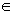U: mA(x)a}, a in [0, 1], are closed real intervals) and whose membership function y=mA (x) is a piecewise continuous function.

For general facts on FNs we refer to the book of Kaufmann and Gupta 15.

A TFN (a, b, c), with a, b, c real numbers such that a < b < c is the simplest form of a FN. It represents mathematically the fuzzy statement “the value of b lies in the interval [a, c]”. The membership function y = m(x) of (a, b, c) is zero outside the interval [a, c], while its graph in [a, c] consists of two straight line segments forming a triangle with the OX axis (Figure 3).

• Figure 3. Graph and Centre of Gravity (CoG) of the TFN (a, b, c)

Therefore we have: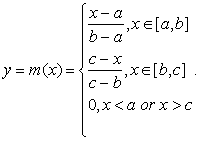Using elementary methods of Analytic Geometry it is straightforward to check ( 2, Proposition 8) that the coordinates (X, Y) of the Centre of Gravity (CoG) of the graph of a TFN A = (a, b, c), being the intersection point G of the medians of the corresponding triangle (Figure 3), are calculated by the formulas(1)

The first of formulas (1) can be used to defuzzify the TFN A with the CoG defuzzification technique 16, i.e. to represent it by a crisp number.

There are two general methods known, equivalent to each other, for defining arithmetic operations on FNs 15. Those methods lead to the following simple rules for the addition and subtraction of TFNs.

Let A=(a, b, c) and B=(a1, b1, c1) be two TFNs. Then one defines:

Ÿ The sum A + B = (a+a1, b+b1, c+c1).

Ÿ The difference A-B=A + (-B)=(a-c1, b-b1, c-a1), where-B=(-c1, -b1, -a1) is defined to be the opposite of B.

On the contrary, the product and the quotient of A and B are FNs, which are not TFNs in general, apart from some special cases.

The following two scalar operations can also be defined:

Ÿ k + A= (k+a, k+b, k+c), kR.

Ÿ kA = (ka, kb, kc), if k>0 and kA = (kc, kb, ka), if k<0, kR.

3.2. The Assessment Method Using TFNs

For the better understanding of the present work our assessment method using TFNs developed in earlier works ( 2, Chapter 7) is recalled here in brief.

For this, let Ai, i = 1, 2, n be given TFNs, where n is a non negative integer, n2. Then, we define the mean value of the Ai’s to be the TFN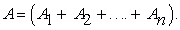The qualitative grades A = excellent, B = very good, C=good, D = fair and F = unsatisfactory are considered for the assessment of a system’s performance. A scale of numerical scores from 1-100 is assigned to them as follows: A (85-100), B (75-84), C (60-74), D (50-59) and F (0-49)1.

Then, each of the above grades can be represented by a TFN, denoted for simplicity by the same letter, as follows: A=(85, 92.5, 100), B=(75, 79.5, 84), C (60, 67, 74), D (50, 54.5, 59) and F (0, 24.5, 49). The middle entry of each of the above TFNs is equal to the average of its other two entries. In other words, if A (a1, b1, c1), B (a2, b2, c2), F (a5, b5, c5), then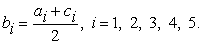In order to assess the total system’s effectiveness, the performance of each of the system’s components is evaluated by one of the above five qualitative grades. This means that one of the TFNs A, B, C, D, F can be assigned to each of those components.

Let n be the total number of the system’s components and let nX be the number of the components corresponding to the TFN X, where X = A, B, C, D, F. Then the mean value M of all those TFNs is equal to the TFN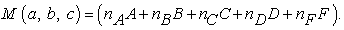(2)

Since the calculation of the mean value of the qualitative grades is not possible, it looks logical to consider the TFN M as a fuzzy representative for evaluating the system’s mean performance. Replacing the values of the TFNs A, B, C, D and F in equation (2) and making the required calculations it is straightforward to check that for M (a, b, c) we have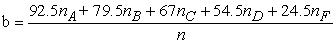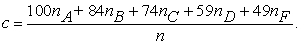Then, equation (1) gives that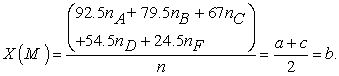(3)

The value of X (M) provides a crisp representation of the TFN M evaluating the system’s mean performance.

3.3. Grey Numbers

Frequently in the everyday life, as well as in many applications of science and engineering, a system’s data cannot be easily determined precisely and in practice estimates of them are used. The reason for this is that in large and complex systems, like the socio-economic, the biological ones, etc., many different and constantly changing factors are usually involved, the relationships among which are indeterminate. This makes their operation mechanisms to be not clear. Two are the main tools today for handling such approximate data: Fuzzy Logic (FL), which is based on the notion of FS initiated by Zadeh 17 in 1965 and the theory of Grey System (GS) initiated by Deng 18 in 1982.

The GS theory was mainly developed in China and it has found nowadays many important applications in agriculture, economy, management, industry, ecology, environment, meteorology, geography, geology, forecast of earthquakes, history, military affairs, sports, traffic, material science, biological protection and in many other fields of the human activity; see 19 and its relevant references.

The systems which lack information, such as structure message, operation mechanism and behaviour document, are referred to as GSs. For example, the human body, the world economy, etc., are GSs. Usually, on the grounds of existing grey relations and elements one can identify where "grey" means poor, incomplete, uncertain, etc.

The aim of the GS theory is to provide techniques, notions and ideas for analyzing latent and intricate systems. For example, the establishment of non-function models, the development of a grey process replacing an existing stochastic process, the transformation of disorderly raw data into a more regular series by grey generating techniques, grey decision making, grey forecasting control, the study of feeling and emotion functions and of fields with whitening functions, etc. 19.

An effective tool for handling the approximate data of a GS is the use of GNs. A GN is an indeterminate number whose probable range is known, but which has unknown position within its boundaries. The GNs are defined with the help of the closed real intervals. More explicitly, if R denotes the set of real numbers, a GN, say A, can be expressed mathematically by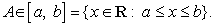If a b, then A is called a white number and if, then A is called a black number. A GN may enrich its uncertainty representation with respect to the interval [a, b] by a whitening function f: [a, b] [0, 1] defining a degree of greyness g(x) for each x in [a, b]. The closer is g(x) to 1, the greater the probability for x to be the representative real value of the corresponding GN. For general facts on GNs we refer to 20.

The well known arithmetic of the real intervals 21 has been used to define the basic arithmetic operations among the GNs. More explicitly, ifandare given GNs and k is a real number, one defines:

Ÿ Addition by A + B[a1 + b1, a2 + b2]

Ÿ Subtraction by A-B = A + (-B)[a1-b2, a2-b1], where-B[-b2, -b1] is defined to be the opposite of B.

Ÿ Multiplication by A x B[min {a1b1, a1b2, a2b1, a2b2}, max {a1b1, a1b2, a2b1, a2b2}].

Ÿ Division bywith b1, b20 and B-1, which is defined to be the inverse of B.

Ÿ Scalar multiplication by kA[ka1, ka2], if k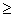0 and by kA[ka2, ka1], if k <0.

Observe that B + (-B)[b1-b2, b2-b1][0, 0], B + (-B)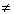(-B) + B0 and B x B-1 = B-1 x B[1, 1].

The white number with the greatest probability to be the representative real value of the GN Aà[a, b] is denoted by W(A). The technique of determining the value of W(A) is called whitening of A.

One usually defines W(A) = (1-t)a + tb, with t in [0, 1]. This is known as the equal weight whitening. If the distribution of A is unknown (i.e. no whitening function has been defined for A), one takes t=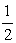, which gives that(4)

### 4. The Assessment Method with GNS

Consider again the numerical scores A (100-85), B (84-75), C (74-60), D (59-50), F (49-0) attached to the corresponding qualitative grades. We correspond to each grade a GN, denoted for simplicity with the same letter, as follows: A∈ [85, 100], B∈[75, 84], C∈ [60, 74], D∈[50, 59] and F∈[0, 49].

Next, assigning to each of the system’s components the GN assessing its individual performance and using the same notation and definitions as in the case of the TFNs, we consider the mean value(5)

of all those GNs as the grey representative of the system’s mean performance.

But nAA[85nA, 100nA], nBB[75nB, 84nB], nCC[60nC, 74nC], nDD[50nD, 59nD] and nFF[0nF, 49nF], therefore it turns out that M[m1, m2], with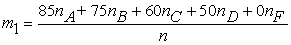andSince the distributions of the GNs A, B, C, D and F are unknown, the same happens with the distribution of M*. Therefore, one can take(6)

From equations (3) and (6) it turns out that X(M)=W(M*), which means that the assessment methods of a system’s mean performance using as tools the TFNs or the GNs A, B, C, D and F respectively are equivalent to each other, providing the same assessment outcomes.

Moreover, one observes that in the extreme case where the maximal possible numerical score corresponds to each component for each grade, i.e. the nA scores corresponding to A are equal to 100, the nB scores corresponding to B are equal to 84, etc., the mean value of all those scores is equal to c or m2 respectively. Also, in the opposite extreme case, where the minimal possible numerical score corresponds to each component for each linguistic grade, i.e. the nA scores corresponding to A are equal to 85, the nB scores corresponding to B are equal to 75, etc., the mean value of all those scores is equal to a or m1 respectively. Therefore, equations (3) and (6) give a reliable approximation of the system’s mean performance. Consequently, the equivalent assessment methods with TFNs and GNs are useful when no numerical scores are used, but the system’s performance is assessed by qualitative grades.

### 5. Examples on the Assessment of CT Skills

As we have seen in Section 2.1, modelling thinking synthesizes all the other modes of thinking involved in CT for constructing a proper model for the solution of the given problem. As a matter of fact, our first example on the assessment of CT skills concerns the evaluation of student mathematical modelling abilities.

Example 1: The following Table depicts the performance of two student groups G1 and G2, in a common mathematical test involving the solution of MM problems:

Assigning to each student the corresponding GN we calculate the mean values M1 and M2 of all those GNs for the groups G1 and G2 respectively, which are approximately equal toandThereforeand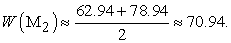Consequently both groups demonstrated a good (C) mean performance, with the mean performance of the second group being slightly better.

A special form of CT systems, with great importance for Artificial Intelligence, are the CBR systems (see Section 2.2) and the following examples concern the evaluation of the effectiveness of such kind of systems.

Example 2: Consider two CBR systems designed for help desk applications with their libraries containing 105 and 90 past cases respectively. Assume that the two systems’ designers have supplied them with the same mechanism (software) that enables the assessment of the degree of success of each one of their past cases at each step of the CBR process, when used for the solution of new similar problems. Table 2 depicts the degree of success of their past cases in each of the three first steps of the CBR process.

We shall compare the quality performance of the two systems by calculating the GPA index and their mean performance by applying our assessment methods with the TFNs and the GNs.

i) GPA index: Denote by yi, i = 1, 2, 3, 4, 5 the ratios of the number of the CBR system’s cases whose performance is characterized by F, D, C, B and A respectively to the total number of its cases (frequencies), then the GPA index is calculated ( 2, Chapter 6, p.125) by the formula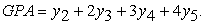(7)

In case of the ideal performance (y5=1) we have GPA=4, while in case of the worst performance (y1=1) we have GPA=0; therefore 0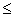GPA4. Consequently, values of GPA greater than 2 could be considered as corresponding to a more than satisfactory system’s performance. In our case, the data of Table 1 give the following frequencies:

Replacing the values of frequencies from Table 3 to formula (7) one finds the following values for the GPA index:

First System: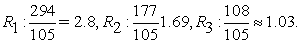Second System:The above values of the GPA index show that the first system demonstrated a better quality performance at steps R1 and R3 (Retrieve, Revise), whereas the second one demonstrated a better performance at R2 (Reuse). Further, the two systems’ performance was proved to be more than satisfactory in R1 and less than satisfactory in the other two steps, being worse at R3. This was logically expected, since the success in each step depends on the success in the previous steps. Notice that the two systems’ performance at the last step R4 was not examined, since ALL the past cases, even the unsuccessful ones, are retained in a system’s library for possible use in future with related new problems; the unsuccessful ones to help for exploring possible reasons of failure to find a solution for the new problem.

Finally, the mean values of the GPA index for the two systems at the three steps R1, R2 and R3 are approximately equal to 1.84 and 1.62 respectively, showing that the first system demonstrated a better overall quality performance.

ii) Use of the TFNs: From the data of Table 2 one finds that for the first system and in step R1 we have 51 TFNs equal to C(60, 67, 74), 24 TFNs equal to B(75, 79.5, 85) and 30 TFNs equal to A(85, 92.5, 100). The mean value of all those TFNs, denoted for simplicity by the same letter R1, is equal to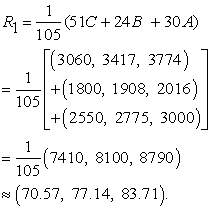Therefore, from equation (3) one gets that X(R1) = 77.14. This shows that the first system demonstrated a very good (B) performance at step R1.

In the same way one calculates for the first system the mean values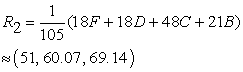andthus obtaining the analogous conclusions for the system’s performance at the steps R2 and R3 of the CBR process.

Finally, the overall system’s performance can be assessed by the mean value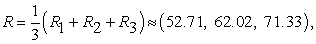Therefore, since X(R) = 62.02, the system demonstrated a good (C) mean performance.

A similar argument gives for the second system the values R1 = (62.5, 68.25, 74), R2(45.33, 55.17, 65), R3 = (33, .46.25, 59.5) and R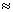(46.94, 56.56, 66.17), thus obtaining the analogous conclusions for its mean performance at each step of the CBR process and its overall mean performance.

iii) Use of the GNs: According to this approach in step R1 we have 51 GNs equal to C∈[60,74], 24 GNs equal to B∈ [75, 84] and 30 GNs equal to A∈ [85, 100]. The mean value of all those GNs, denoted by R1*, is equal to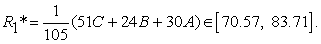Therefore,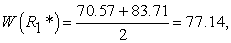etc.

As we have seen in Section 4 this approach provides in general the same assessment outcomes with the use of TFNs, but, as it becomes more evident from the present example, it reduces significantly the required computational burden.

Table 4 depicts the assessment outcomes obtained in this example.

The outcomes of Table 4 illustrate the fact that the assessment of a system’s quality performance could lead to different outcomes from the assessment of its mean performance (step R2).

Example 3: Six different users of a CBR system ranked with scores from 0-100 the effectiveness of the following five past cases, stored in its case-library, for solving new related problems: C1 (Case 1): 43, 48, 49, 49, 50, 52, C2: 81, 83. 85, 88, 91, 95, C3: 76, 82, 89, 95, 95, 98, C4: 86, 86, 87, 87, 87, 88, C5: 35, 40, 44, 52, 59, 62.

The mean value of the given, 6X5 = 30in total numerical scores is approximately equal to 72.07 demonstrating a good (C) mean performance of the system with respect to the above five past cases. In order to compare the assessment outcomes the system’s mean performance will also be calculated by using our model with the GNs.

In fact, the given numerical scores correspond to 14 GNs equal to the GN A, 4 equal to B, 1 equal to C, 4 equal to D and 7 GNs equal to the GN F. The mean value of the above GNs is equal to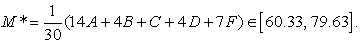Therefore, from equation (6) one obtains that W(M*) = 69.98. Consequently the CBR system demonstrates a good mean performance with respect to the given five past cases. However the exact score corresponding to the system’s mean performance is equal to the mean value 72.02 of the given numerical scores. In concluding, the assessment model using GNs (or TFNs), although it gives a good approximation of the system’s mean performance, it is actually useful only when the effectiveness of the system’s past cases is evaluated by qualitative grades and not by numerical scores, because in this case the calculation of the mean value of those grades is not possible.

Our last example gives a method of solving algebraic systems with grey coefficients.

Example 4: The performance of a CBR system in solving two different problems is represented by the GNs A and B respectively such that A + B[2, 4] and A. B∈[1,3]. It is asked to compare the system’s performance in those two cases to its top possible performance to which the numerical score 5 has being assigned.

Solution: The whitening of the given sum and product of the GNs A and B leads to the solution of the ordinary system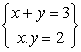, which gives that x=.2, y=1 or vice versa. Therefore W(A) = 2 and W(B) =1 or vice versa, which means that the system’s performance in the above two cases was equal to the 40% and the 20% respectively of its top possible performance.

Remark: The attempt to solve the above problem by applying the definitions of addition and multiplication of GNs presented in Section 3 leads to a system of equations with respect to the unknown numbers a1, b1, a2, b2 which does not provide a concrete solution. For more details on solving systems of equations with grey data see 22.

### 6. Conclusions and Discussion

A method using GNs as tools was developed in the present research for assessing a system’s mean performance, which is useful when using qualitative grades and not numerical scores for this purpose. This new method was proved to be equivalent with an analogous method using TFNs instead of GNs developed in earlier works, but it reduces significantly the required computational burden, since it requires the calculation of two components only (instead of three in case of the TFNs) for obtaining the mean value of the GNs. Examples were also presented on the assessment of CT systems illustrating our results and showing that the system’s quality performance, calculated by the traditional GPA index, may lead to different assessment conclusions.

Although the enormous development of technology during the last years makes easier and more comfortable the human life, it creates in parallel more and more complicated artificial systems, which are difficult to be managed by the traditional scientific methods. As a result, the applications of FL and of the GS theory have been rapidly expanded nowadays covering almost all sectors of the human activities. In particular the FNs and the GNs have been proved to be effective tools in handling approximate and/or uncertain data, playing an important role in fuzzy mathematics and in GS theory respectively, analogous to the role played by the ordinary numbers in crisp mathematics. Therefore, the attempt to use FNs or GNs in other applications, apart from the assessment purposes, to science and technology and to the everyday life looks as a very interesting and promising direction for future research on the subject. Note that such efforts have been already started by the present author on solving equations, systems of equations and linear programming problems with fuzzy or grey data, connected to real life applications 22, 23, 24.

### References

  Balley, K. D., Sociology and the New Systems Theory: Toward a Theoretical Synthesis. New York: State of New York Press, 1994. In article  Voskoglou, M. Gr., Finite Markov Chain and Fuzzy Logic Assessment Models: Emerging Research and Opportunities, Columbia, SC, Createspace.com.-Amazon, 2017. In article  Voskoglou, M. Gr., Use of the Triangular Fuzzy Numbers for Student Assessment, American Journal of Applied Mathematics and Statistics, 3(4), 146-150, 2015. In article  Green, A. J. K. & Gillhooly, K., Problem solving, in Braisby, N. & Gelatly, A. (Eds.), Cognitive Psychology, Oxford University Press, Oxford, 2005. In article  Mc Peck, J. E., Critical thinking and education, Martin Robinson, Oxford, 1981. In article  Salomon, G. & Perkings, D., Rocky roads to transfer: Rethinking mechanisms of a neglected phenomenon, Educational Psychologist, 24, 113-142, 1989. In article View Article  Mc Guinness, C., Teaching thinking: New signs for theories of cognition, Educational Psychology,, 13(3-4), 305-316, 1993 In article View Article  Papert, S., An exploration in the space of Mathematics Education, International Journal of Computers for Mathematics, 1(1), 95-123, 1996. In article  Wing, J. M., Computational thinking, Communications of the ACM, 49, 33-35, 2006. In article View Article  Voskoglou, M.Gr. & Buckley, S., Problem Solving and Computers in a Learning Environment, Egyptian Computer Science Journal,, 36(4), 28-46, 2012. In article  Liu, J. & Wang, L., Computational Thinking in Discrete Mathematics, Proceedings of the IEEE 2nd International Workshop on Education Technology and Compute Science, pp. 413-416, 2010. In article View Article  Kazimoglu, C., Kiernan, M., Bacon, L. & MacKinnon, L., Understanding Computational Thinking Before Programming: Developing Guidelines for the Design of Games to Learn Introductory Programming through Game-Play, International Journal of Game-Based Learning, 1(3), 30-52, 2011. In article View Article  Voskoglou, M. Gr. & Salem A.-B.M., Analogy-Based and Case-Based Reasoning: Two Sides of the Same Coin, International Journal of Applications of Fuzzy Sets and Artificial Intelligence, 4, 7-18, 2014. In article  Klir, G. J. & Folger, T. A., Fuzzy Sets, Uncertainty and Information, Prentice-Hall, London, 1988. In article  Kaufmann, A. & Gupta, M., Introduction to Fuzzy Arithmetic, Van Nostrand Reinhold Company, New York, 1991. In article  Van Broekhoven, E. & De Baets, B., Fast and accurate centre of gravity defuzzification of fuzzy systems outputs defined on trapezoidal fuzzy partitions, Fuzzy Sets and Systems, 157(7), 904-918, 2006. In article View Article  Zadeh, L.A., Fuzzy Sets, Information and Control, 8, 338-353, 1965. In article View Article  Deng, J., Control Problems of Grey Systems, Systems and Control Letters, 288-294, 1982. In article  Deng, J., Introduction to Grey System Theory, The Journal of Grey System, 1, 1-24, 1989. In article  Liu, S. F. & Lin, Y. (Eds.), Advances in Grey System Research, Berlin-Heidelberg: Springer, 2010. In article View Article  Moore, R.A., Kearfort, R.B. & Clood, M.J., Introduction to Interval Analysis, 2nd Printing, Philadelphia, SIAM, 1995. In article  Voskoglou, M. Gr., Solving Systems of Equations with Grey Data, International Journal of Applications of Fuzzy Sets and Artificial Intelligence, 8, 103-111, 2018. In article  Voskoglou, M. Gr., Solving Linear Programming Problems with Grey Data, Oriental Journal of Physical Sciences, 3(1), 17-23, 2018. In article  Voskoglou, M. Gr., Fuzzy Linear Programming, Egyptian Journal of Computer Science, 42(3), 1-14, 2018. In articleThis work is licensed under a Creative Commons Attribution 4.0 International License. To view a copy of this license, visit http://creativecommons.org/licenses/by/4.0/